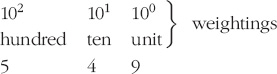Representing and manipulating data in computers

Start this free course now. Just create an account and sign in. Enrol and complete the course for a free statement of participation or digital badge if available.

Free course

# 2.2.1 Positive integers: denary numbers

The number system which we all use in everyday life is called the denary representation, or sometimes the decimal representation, of numbers. In this system, the ten digits 0 to 9 are used, either singly or in ordered groups. The important point for you to grasp is that when the digits are used in ordered groups, each digit is understood to have a weighting. For example, consider the denary number 549. Here 5 has the weighting of hundreds, 4 has the weighting of tens and 9 has the weighting of units. (Try saying 549 aloud: five hundred and forty nine. If you remember that ‘ty’ is a corruption of ‘ten’ you can see that the way the number is said is exactly consistent with this idea of weightings.)So 549 stands for:

(5 × 102) + (4 × 101) + (9 × 100)

which is:

(5 × 100) + (4 × 10) + (9 × 1)

In the foregoing, the raised numbers after the various tens are called exponents. If you are unfamiliar with exponent notation, you should note that 102 means 10 x 10, 103 means 10 x 10 x 10, and so on. 101 simply means 10 and 10° is taken to be 1. There is more about this in the Numeracy Resource (click on the link below) if it's an unfamiliar idea to you.

Please click on the 'View document' link below to view the Numeracy Resource.

View document [Tip: hold Ctrl and click a link to open it in a new tab. (Hide tip)]

The position of the digit in the group is therefore crucial in determining its weighting. The leftmost digit in the group (5 in the above example) is called the most-significant digit because it is the most heavily weighted digit. Similarly, the rightmost digit (9 above) is called the least-significant digit.

A number representation like this, where a digit's position determines its weighting, is called a positional notation. Notice the pattern of the exponents; they increase by 1 from right to left.

## Activity 2 (Self assessment)

In the denary number 10 276:

1. What are the weightings of

1. the 7

2. the 0

3. the 1?

2. What is the most-significant digit?

3. Write out this number in a similar way to the way 549 was written out in the preceding text – that is, as a sum of terms of the form (digit × 10 to some exponent).

1.

1. tens (the 7 represents 70)

2. thousands (there are no thousands)

3. ten thousands (the 1 represents 10 000)

2. 1

3. (1 × 104) + (0 × 103) + (2 × 102) + (7 × 101) + (6 × 100)